Question

# Selected sales and operating data for three divisions of different structural engineering firms are given as...

Selected sales and operating data for three divisions of different structural engineering firms are given as follows: Division A Division B Division C Sales \$ 6,800,000 \$ 10,800,000 \$ 9,900,000 Average operating assets \$ 1,360,000 \$ 2,700,000 \$ 1,980,000 Net operating income \$ 401,200 \$ 1,069,200 \$ 311,850 Minimum required rate of return 23.00 % 39.60 % 20.00 % Required:

1. Compute the return on investment (ROI) for each division using the formula stated in terms of margin and turnover.

2. Compute the residual income (loss) for each division.

3. Assume that each division is presented with an investment opportunity that would yield a 25% rate of return.

a. If performance is being measured by ROI, which division or divisions will probably accept or reject the opportunity?

b. If performance is being measured by residual income, which division or divisions will probably accept or reject the opportunity?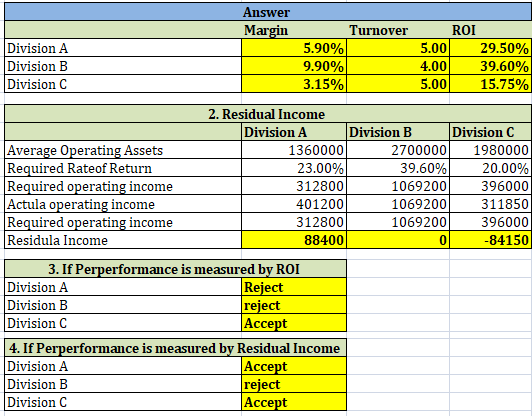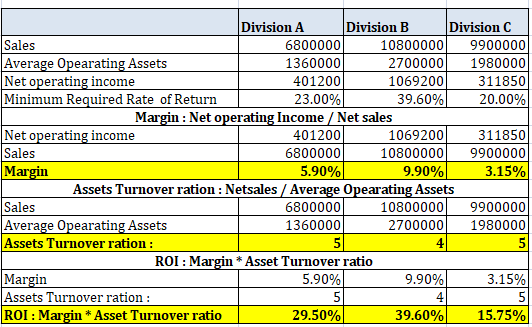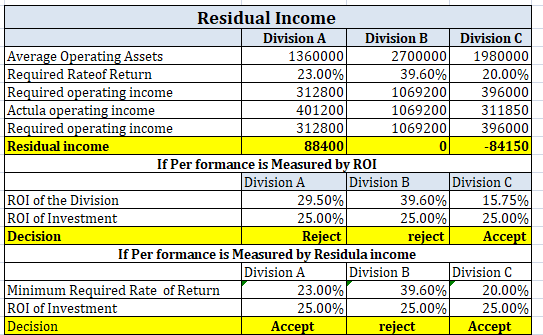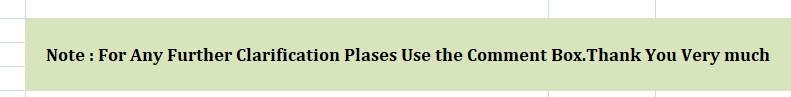#### Earn Coins

Coins can be redeemed for fabulous gifts.

Similar Homework Help Questions
• ### Selected sales and operating data for three divisions of different structural engineering firms are given as...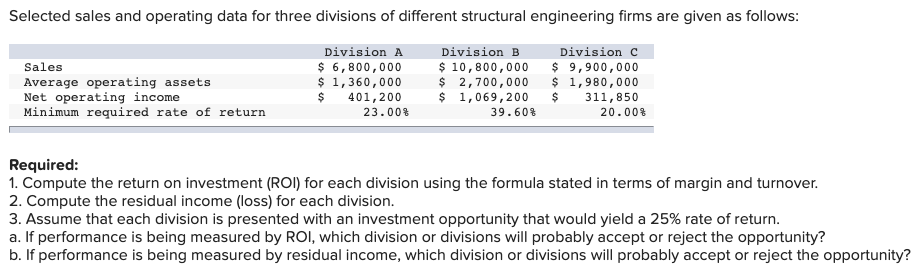Selected sales and operating data for three divisions of different structural engineering firms are given as follows: Sales Average operating assets Net operating income Minimum required rate of return Division A \$ 6,800,000 \$ 1,360,000 \$ 401,200 23.00% Division B \$ 10, 800,000 \$ 2,700,000 \$ 1,069,200 39.60% \$ 9,900,000 \$ 1,980,000 \$ 311,850 20.00% Required: 1. Compute the return on investment (ROI) for each division using the formula stated in terms of margin and turnover. 2. Compute the residual...

• ### Selected sales and operating data for three divisions of different structural engineering firms are given as...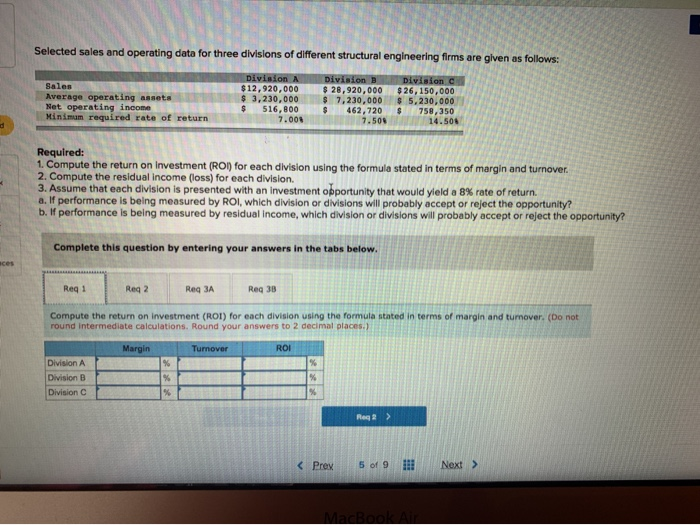Selected sales and operating data for three divisions of different structural engineering firms are given as follows: Sales Average operating assets Net operating income Minimum required rate of return Division \$12,920,000 \$ 3.230.000 \$ 516.800 7.000 Division \$ 28,920,000 \$ 7,230,000 462.720 7.500 Division \$26,150,000 \$5,230,000 758.350 14.50 Required: 1. Compute the return on investment (ROI) for each division using the formula stated in terms of margin and turnover. 2. Compute the residual income (loss) for each division 3. Assume...

• ### Selected sales and operating data for three divisions of different structural engineering firms are given as...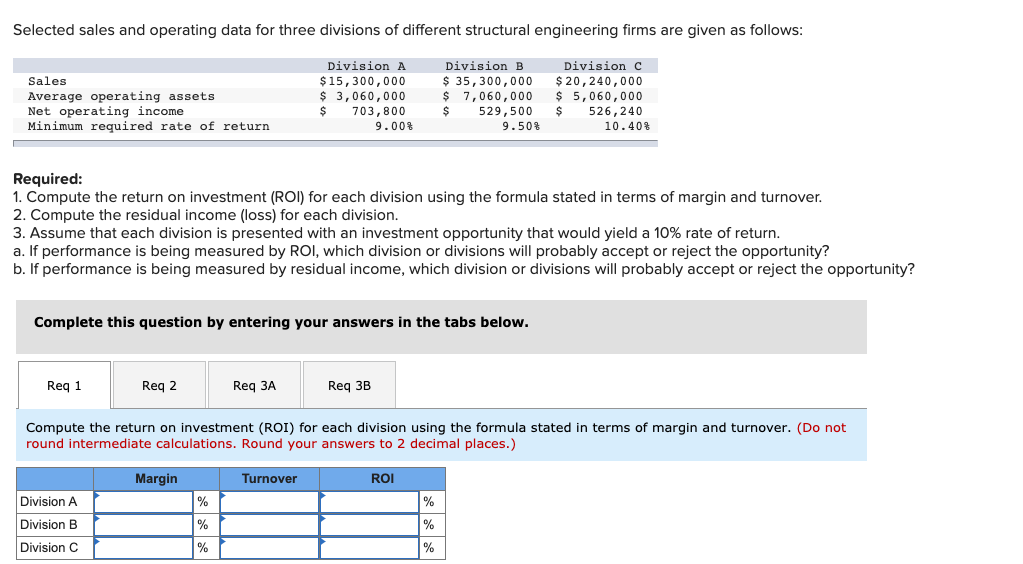Selected sales and operating data for three divisions of different structural engineering firms are given as follows: Sales Average operating assets Net operating income Minimum required rate of return Division A \$ 15,300,000 \$ 3,060,000 \$ 703,800 9.00% Division B \$ 35,300,000 \$ 7,060,000 \$ 529,500 9.50% Division C \$20, 240,000 \$ 5,060,000 \$ 526,240 10.40% Required: 1. Compute the return on investment (ROI) for each division using the formula stated in terms of margin and turnover. 2. Compute the...

• ### Selected sales and operating data for three divisions of different structural engineering firms are given as...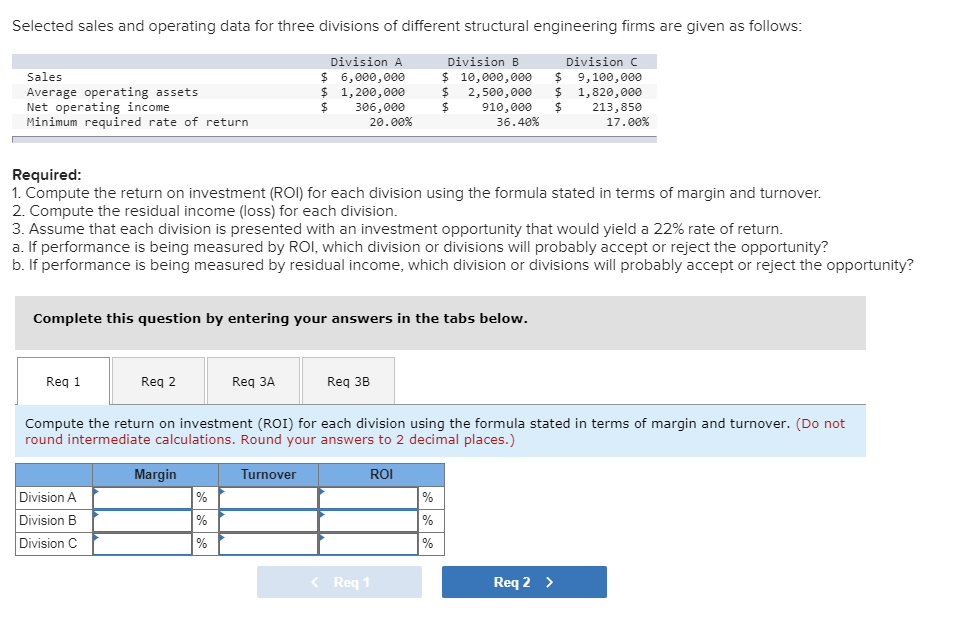Selected sales and operating data for three divisions of different structural engineering firms are given as follows: Division A Division B Division C Sales \$ 6,000,000 \$ 10,000,000 \$ 9,100,000 Average operating assets \$ 1,200,000 \$ 2,500,000 \$ 1,820,000 Net operating income \$ 306,000 \$ 910,000 \$ 213,850 Minimum required rate of return 20.00 % 36.40 % 17.00 % Required: 1. Compute the return on investment (ROI) for each division using the formula stated in terms of margin and turnover....

• ### Selected sales and operating data for three divisions of different structural engineering firms are given as...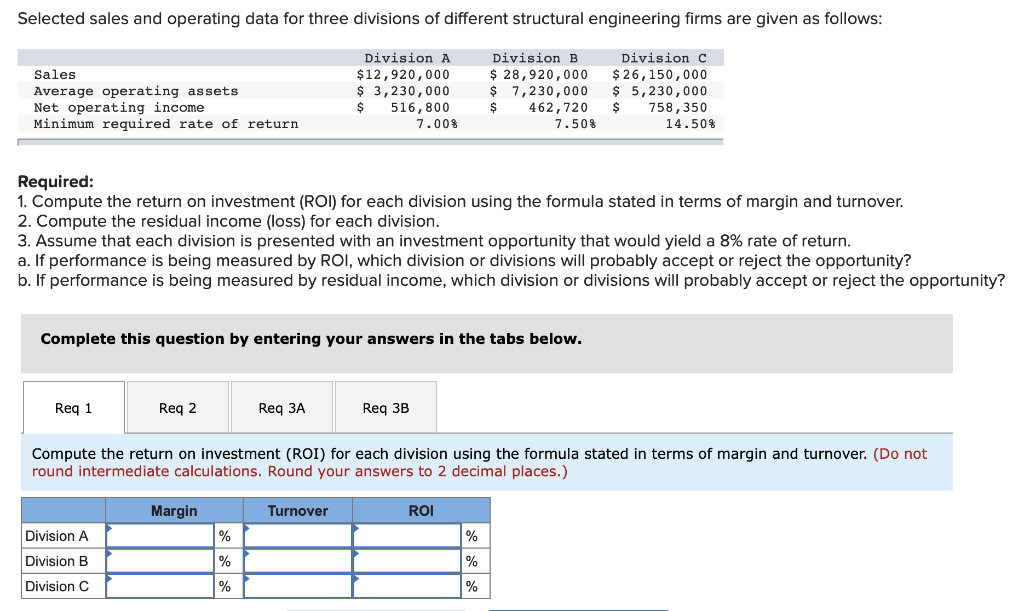Selected sales and operating data for three divisions of different structural engineering firms are given as follows: Division A Division B Division C Sales \$ 12,920,000 \$ 28,920,000 \$ 26,150,000 Average operating assets \$ 3,230,000 \$ 7,230,000 \$ 5,230,000 Net operating income \$ 516,800 \$ 462,720 \$ 758,350 Minimum required rate of return 7.00 % 7.50 % 14.50 % Required: 1. Compute the return on investment (ROI) for each division using the formula stated in terms of margin and turnover....

• ### Selected sales and operating data for three divisions of different structural engineering firms are given as...

Selected sales and operating data for three divisions of different structural engineering firms are given as follows: Division A Division B Division C Sales \$ 15,050,000 \$ 35,050,000 \$ 25,050,000 Average operating assets \$ 3,010,000 \$ 7,010,000 \$ 5,010,000 Net operating income \$ 617,050 \$ 525,750 \$ 701,400 Minimum required rate of return 9.00 % 9.50 % 14.00 % Required: 1. Compute the return on investment (ROI) for each division using the formula stated in terms of margin and turnover....

• ### Selected sales and operating data for three divisions of different structural engineering firms are given as...

Selected sales and operating data for three divisions of different structural engineering firms are given as follows: Division A Division B Division C Sales \$ 6,900,000 \$ 10,900,000 \$ 10,000,000 Average operating assets \$ 1,725,000 \$ 5,450,000 \$ 2,500,000 Net operating income \$ 414,000 \$ 1,090,000 \$ 325,000 Minimum required rate of return 19.00 % 20.00 % 16.00 % Required: 1. Compute the return on investment (ROI) for each division using the formula stated in terms of margin and turnover....

• ### Selected sales and operating data for three divisions of different structural engineering firms are given as...

Selected sales and operating data for three divisions of different structural engineering firms are given as follows: Division A Division B Division C Sales \$ 5,700,000 \$ 9,700,000 \$ 8,800,000 Average operating assets \$ 1,140,000 \$ 4,850,000 \$ 1,760,000 Net operating income \$ 273,600 \$ 853,600 \$ 180,400 Minimum required rate of return 17.00 % 17.60 % 14.00 % Required: 1. Compute the return on investment (ROI) for each division using the formula stated in terms of margin and turnover....

• ### Selected sales and operating data for three divisions of different structural engineering firms are given as...

Selected sales and operating data for three divisions of different structural engineering firms are given as follows: Division A Division B Division C Sales \$ 6,500,000 \$ 10,500,000 \$ 9,600,000 Average operating assets \$ 1,300,000 \$ 5,250,000 \$ 1,920,000 Net operating income \$ 364,000 \$ 1,008,000 \$ 273,600 Minimum required rate of return 21.00 % 19.20 % 18.00 % Required: 1. Compute the return on investment (ROI) for each division using the formula stated in terms of margin and turnover....

• ### Selected sales and operating data for three divisions of different structural engineering firms are given as...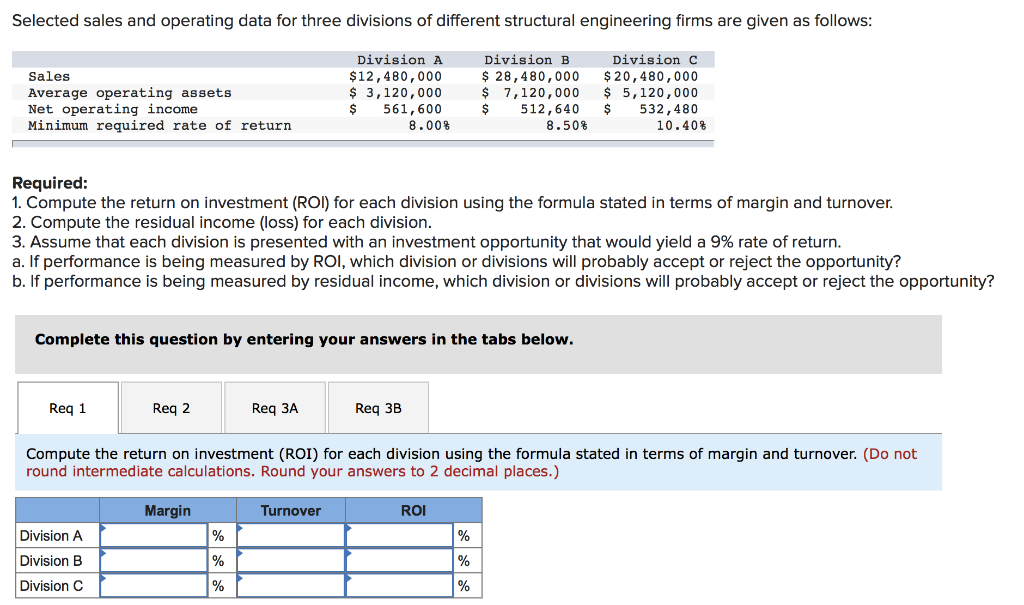Selected sales and operating data for three divisions of different structural engineering firms are given as follows: Sales Average operating assets Net operating income Minimum required rate of return Division A \$12,480,000 \$ 3,120,000 \$ 561,600 8.008 Division B \$ 28,480,000 \$ 7,120,000 \$ 512,640 8.50% Division c \$ 20,480,000 \$ 5,120,000 \$ 532,480 10.40% Required: 1. Compute the return on investment (ROI) for each division using the formula stated in terms of margin and turnover. 2. Compute the residual...